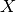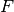# Functional¶

class `odl.solvers.functional.functional.``Functional`(*args, **kwargs)[source]

Implementation of a functional class.

A functional is an operator `f` that maps from some domain `X` to the field of scalars `F` associated with the domain:

`f : X -> F`.

Notes

The implementation of the functional class assumes that the domainis a Hilbert space and that the field of scalarsis a is the real numbers. It is possible to create functions that do not fulfil these assumptions, however some mathematical results might not be valid in this case. For more information, see the ODL functional guide.

Attributes
`adjoint`

Adjoint of this operator (abstract).

`convex_conj`

Convex conjugate functional of the functional.

`domain`

Set of objects on which this operator can be evaluated.

`grad_lipschitz`

Lipschitz constant for the gradient of the functional.

`gradient`

Gradient operator of the functional.

`inverse`

Return the operator inverse.

`is_functional`

`True` if this operator’s range is a `Field`.

`is_linear`

`True` if this operator is linear.

`proximal`

Proximal factory of the functional.

`range`

Set in which the result of an evaluation of this operator lies.

Methods

 `_call`(self, x[, out]) Implementation of the operator evaluation. `bregman`(self, point, subgrad) Return the Bregman distance functional. `derivative`(self, point) Return the derivative operator in the given point. `norm`(self[, estimate]) Return the operator norm of this operator. `translated`(self, shift) Return a translation of the functional.
`__init__`(self, space, linear=False, grad_lipschitz=nan)[source]

Initialize a new instance.

Parameters
space`LinearSpace`

The domain of this functional, i.e., the set of elements to which this functional can be applied.

linearbool, optional

If `True`, the functional is considered as linear.

grad_lipschitzfloat, optional

The Lipschitz constant of the gradient. Default: `nan`